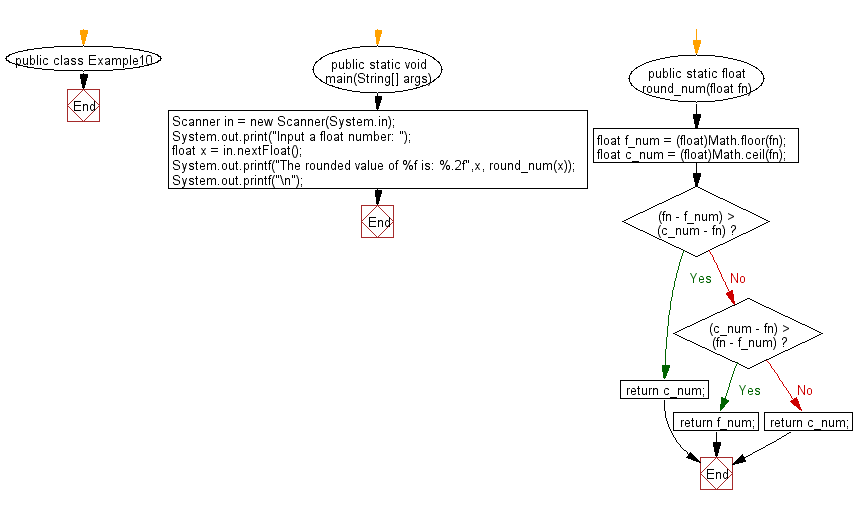﻿ Java Math Exercises: Accept a float value of number and return a rounded float value - w3resource# Java Math Exercises: Accept a float value of number and return a rounded float value

## Java Math Exercises: Exercise-10 with Solution

Write a Java program to accept a float value of number and return a rounded float value.
Sample data:
Input a float number: 12.51
The rounded value of 12.510000 is: 13.00
Input a float number: 12.49999
The rounded value of 12.499990 is: 12.00

Sample Solution:

Java Code:

``````import java.util.*;
public class Example10 {

public static void main(String[] args)
{
Scanner in = new Scanner(System.in);
System.out.print("Input a float number: ");
float  x = in.nextFloat();
System.out.printf("The rounded value of %f is: %.2f",x, round_num(x));
System.out.printf("\n");
}

public static float round_num(float fn)
{
float f_num = (float)Math.floor(fn);
float c_num = (float)Math.ceil(fn);
if ((fn - f_num) > (c_num - fn))
{
return c_num;
}
else if ((c_num - fn) > (fn - f_num))
{
return f_num;
}
else
{
return c_num;
}
}

}
``````

Sample Output:

```Input a float number: 12.53
The rounded value of 12.530000 is: 13.00
```

Flowchart:Java Code Editor:

What is the difficulty level of this exercise?

﻿

## Java: Tips of the Day

Parsing dates:

```import java.io.*;
import java.util.*;
import java.text.*;

String s = "2001/09/23 14:39";

SimpleDateFormat formatter = new SimpleDateFormat ("yyyy/MM/dd H:mm");
Date d = formatter.parse(s, new ParsePosition(0));
```